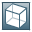Show TOC##enum RFCTYPE### Use

Defines RFC parameter types for each corresponding ABAP types.

### Structure

typedef enum _RFCTYPE

{

RFCTYPE_CHAR   = 0,      /* 1-byte or multibyte character, fixed sized, blank padded */

RFCTYPE_DATE   = 1,      /* date ( YYYYYMMDD ) */

RFCTYPE_BCD    = 2,      /* packed number, any length between 1 and 16 bytes */

RFCTYPE_TIME   = 3,      /* time (HHMMSS)  */

RFCTYPE_BYTE   = 4,      /* raw data, binary, fixed length, zero padded.*/

RFCTYPE_TABLE   = 5,     /* internal table */

RFCTYPE_FLOAT  = 7,      /* floating point, double precission */

RFCTYPE_INT    = 8,      /* 4-byte integer */

RFCTYPE_INT2   = 9,      /* 2-byte integer, obsolete, not directly supported by ABAP/4 */

RFCTYPE_INT1   = 10,     /* 1-byte integer, obsolete, not directly supported by ABAP/4 */

RFCTYPE_NULL  = 14,      /* not supported data type. */

RFCTYPE_STRUCTURE = 17,  /* abap structure */

RFCTYPE_DECF16  = 23,      /* 16-bytes decimal float point */

RFCTYPE_DECF32  = 24,      /* 32-bytes decimal float point */

RFCTYPE_XMLDATA = 28,      /* variable-length, zero terminated string representing ABAP data   with hierarchical structure (so-called TYPE2) as XML */

RFCTYPE_STRING = 29,      /* variable-length, zero terminated string */

RFCTYPE_XSTRING = 30,     /* variable-length raw string, length in bytes */

RFCTYPE_EXCEPTION = 98,

_RFCTYPE_max_value      /* the max. value of RFCTYPEs */

}RFCTYPE;